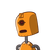# find number are in ratio 1 2 3 and sum of their cubes is 4500​

find number are in ratio 1 2 3 and sum of their cubes is 4500​

### 1 thought on “find number are in ratio 1 2 3 and sum of their cubes is 4500​”

1.5, 10 and 15

Step-by-step explanation:

a = k

b = 2k

c = 3k

Given,

a³ + b³ + c³ = 4500

So,

(k)³ + (2k)³ + (3k)³ = 4500

k³ + 8k³ + 27k³ = 4500

36k³ = 4500

k³ = 125

k = 5

Therefore,

a = k = 5

b = 2k = 10

c = 3k = 15